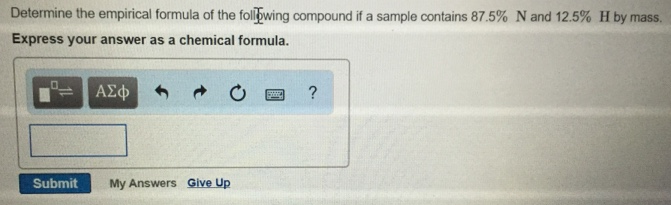# Problem: Determine the empirical formula of the following compound if a sample contains 87.5% N and 12.5% H by mass. Express your answer as a chemical formula.

###### FREE Expert Solution
83% (64 ratings)###### Problem Details

Determine the empirical formula of the following compound if a sample contains 87.5% N and 12.5% H by mass.